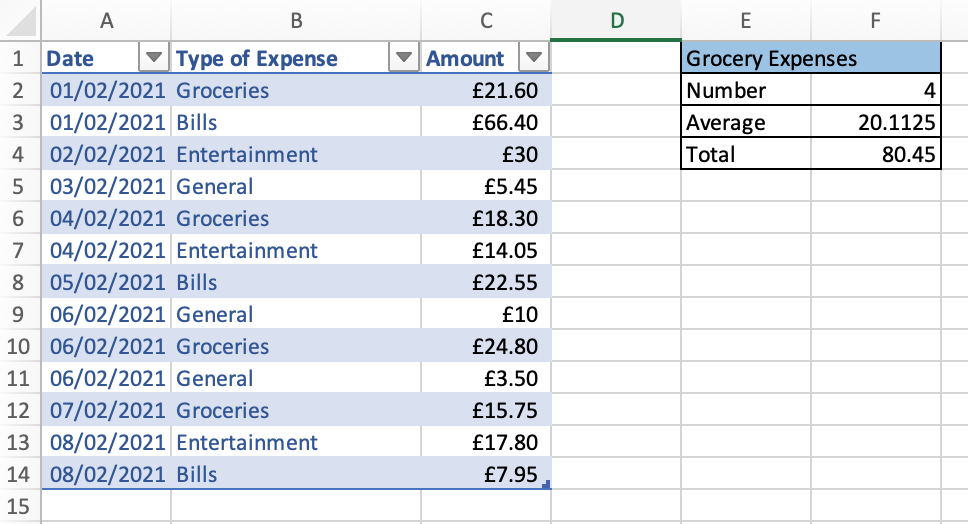### DEV4 Blog

• Home
• /
• Blog
• /
• Microsoft Excel: COUNTIF, AVERAGEIF and SUMIF

### Microsoft Excel: COUNTIF, AVERAGEIF and SUMIF

COUNTIF, AVERAGEIF and SUMIF are three Excel functions which help ease the process of analysing data.

COUNTIF counts how many of the data cells meet the conditions specified. In the example below we will use COUNTIF to count how many times the expense is labelled as “Groceries”. The formula for COUNTIF is =COUNTIF(cell range to search, conditions to search for), e.g., in the below example we use =COUNTIF(B2:B14, “Groceries).

AVERAGEIF allows us to find the average value of the data from cells which meet the search conditions. In our example, we use AVERAGEIF to find out the average “Groceries” expense. The formula used is =AVERAGEIF(cell range to search for specific conditions, conditions to search for, cell range for which we want to find the average), e.g., below we used =AVERAGEIF(B2:B14, “Groceries”, C2:C14).

SUMIF allows you to add up all the data from data cells which meet the conditions specified. In the below example, we use SUMIF to add up the total for expenses labelled as “Groceries”. The formula used would be =SUMIF(cell range to search for specified conditions, conditions to search for, cell range for which we want to find the sum), e.g., below we use =SUMIF(B2:B14, “Groceries”, C2:C14) to see the sum of total grocery expenses.Published on 10 Mar 2021 by Joanna Hepburn